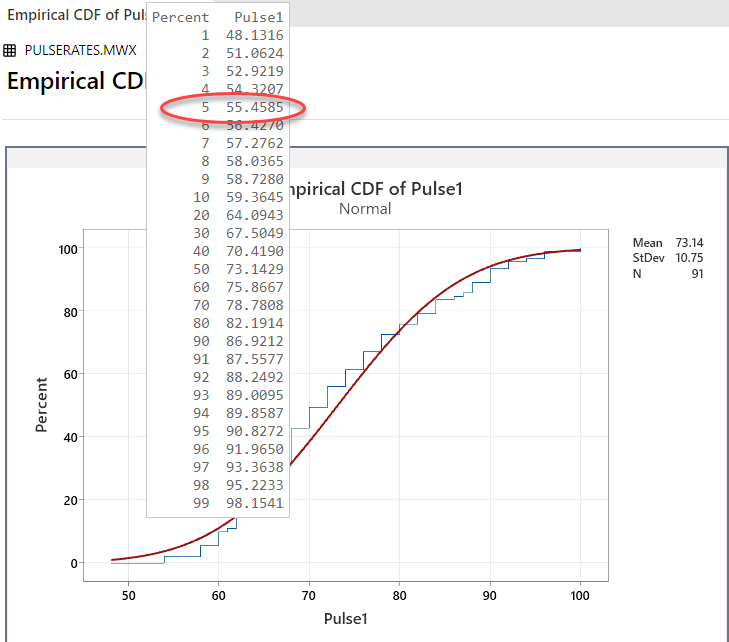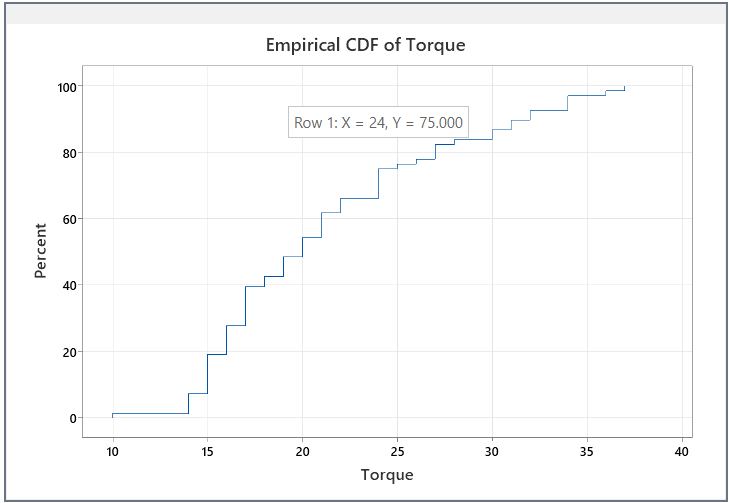# Interpret the key results for Empirical CDF Plot

Complete the following steps to interpret an empirical CDF plot.

## Step 1: Assess the fit of the distribution

The fitted distribution line represents a distribution with parameters estimated from your sample. Assess how closely the fitted distribution line follows the stepped empirical cumulative distribution line. If the stepped line follows the fitted distribution line closely, then the data fits the distribution well.

For a better indication of the distribution fit, use a probability plot to assess the fit for statistical significance. For information on options for fitted distribution lines, go to Fitted distribution lines.

## Step 2: Display estimated percentiles for the population

Hold your pointer over the fitted distribution line to see a chart of percentiles and values.

For example, the following empirical CDF plot shows the pulse rates of test subjects as they walked on a treadmill. For a normal distribution with a mean and standard deviation equal to the data, we would expect 5% of the population to have a pulse rate of 54.7619 or less.###### Note

The estimated population percentiles are accurate only if the data follow the distribution closely.

## Step 3 (optional): Display actual percentiles for the sample data

If your data do not follow the distribution for the cumulative distribution fit line, you can view the actual percentiles for the data values in your sample. First, delete the cumulative distribution fit line because the distribution does not apply. To delete the distribution fit line, right-click the graph and choose Select Item > Distribution Fit, then press the Delete key. Then, move your pointer along the connect line to see tooltips that show individual values (x) and their corresponding percentiles (y).
###### CAUTION

The actual percentiles indicate the percentage of data in your sample, not the population. Therefore, you may not be able to draw general inferences from these results, especially if the sample size is not large. The more data you have to define a percentile, the more accurately the sample percentile will represent your process.

This empirical CDF plot shows the torque required to open caps of shampoo bottles. The tooltip shows that approximately 75% of the caps in the sample required a torque of 24 or less to open.###### Tip

Add gridlines or reference lines to better estimate locations along the connect line. Right-click the graph and choose Add > Gridlines or Add > Reference Lines.

By using this site you agree to the use of cookies for analytics and personalized content.  Read our policy